Module 14 - Answers Lesson 1 Answer 1 14.1.1   The bottom of the ladder moves at a constant rate of 3 ft/sec while the top of the ladder falls at an increasing rate.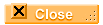Answer 2 14.1.2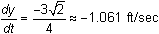. The negative sign indicates that y is decreasing.Answer 3 14.1.3   The velocity of the ladder's top is given by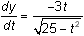Answer 4 14.1.4   Solving yt2(t)=0 indicates that the top of the ladder hits the ground after 5 seconds, butis undefined at that time. As t approaches 5 seconds,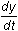grows without bound. So in theory the top is going infinitely fast when it hits the ground as suggested by the following figure.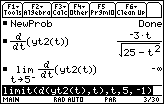Lesson 2 Answer 1 14.2.1 The parametric equations that model the ladder's position at time t are shown in the screen below.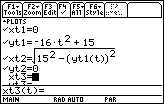Answer 2 14.2.2 The top of the ladder is falling at an increasing rate while the bottom is moving at a decreasing rate.Answer 3 14.2.3 Using the derivative of the position of the bottom of the ladder, xt2(t), and evaluating it at the given times, the bottom is moving at approximately 21.2015 ft/sec, 17.2582 ft/sec, and 8.5665 ft/sec, respectively.Lesson 3 Answer 1 14.3.1 About 61.0 nautical miles.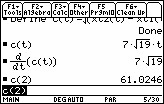Answer 2 14.3.2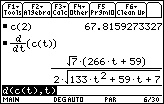Self Test Answer 1 x(t) = 0 y(t) = 15 – 45t Answer 2 x(t) = 24 – 52t y(t) = 0 Answer 3 The car coming from the North will arrive at the intersection first. Answer 4 Define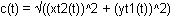Answer 5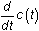with t = 0.25 is approximately -63.739, so the distance between the cars is decreasing at a rate of 63.739 mph.©Copyright 2007 All rights reserved. | Trademarks | Privacy Policy | Link Policy